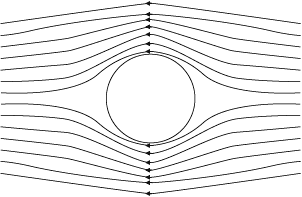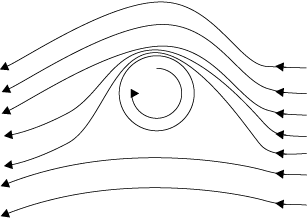# Dynamic Lift

Dynamic lift is the force that acts on a body, such as airplane wing, a hydrofoil or a spinning ball, by virtue of its motion through a fluid. In many games such as cricket, tennis, baseball, or golf, we notice that a spinning ball deviates from its parabolic trajectory as it moves through air. This deviation can be partly explained on the basis of Bernoulli’s principle.

(i) Ball moving without spin: Fig. 1a shows the streamlines around a non-spinning ball moving relative to a fluid. From the symmetry of streamlines it is clear that the velocity of fluid (air) above and below the ball at corresponding points is the same resulting in zero pressure difference. The air therefore, exerts no upward or downward force on the ball.Fig.1a

(ii) Ball moving with spin: A ball which is spinning drags air along with it. If the surface is rough more air will be dragged. Fig 1(b) shows the streamlines of air for a ball which is moving and spinning at the same time. The ball is moving forward and relative to it the air is moving backwards. Therefore, the velocity of air above the ball relative to the ball is larger and below it is smaller (see Section 10.3). The stream lines, thus, get crowded above and rarified below.Fig.1b

This difference in the velocities of air results in the pressure difference between the lower and upper faces and there is a net upward force on the ball. This dynamic lift due to spining is called Magnus effect. Aerofoil or lift on aircraft wing: Figure 1.

(c) shows an aerofoil, which is a solid piece shaped to provide an upward dynamic lift when it moves horizontally through air. The cross-section of the wings of an aeroplane looks somewhat like the aerofoil shown in Fig. 1(c) with streamlines around it. When the aerofoil moves against the wind, the orientation of the wing relative to flow direction causes the streamlines to crowd together above the wing more than those below it. The flow speed on top is higher than that below it. There is an upward force resulting in a dynamic lift of the wings and this balances the weight of the plane. The following example illustrates this.Fig.1c

Example 1

A fully loaded Boeing aircraft has a mass of 3.3 × 10$^5$  kg. Its total wing area is 500 m$^2$. It is in level flight with a speed of 960 km/h.

(a) Estimate the pressure difference between the lower and upper surfaces of the wings

(b) Estimate the fractional increase in the speed of the air on the upper surface of the wing relative to the lower surface. [The density of air is ρ = 1.2 kg.m$^{-3}$]

(a) The weight of the Boeing aircraft is balanced by the upward force due to the pressure difference

∆P × A = 3.3 × 10$^5$ kg × 9.8 m.s$^{-2}$

∆P = (3.3 × 105 kg × 9.8 m.s$^{-2}$)/500 m$^2$

= 6.5 × 10$^3$  N.m$^{-2}$

(b) We ignore the small height difference between the top and bottom sides in Eq. (10.12). The pressure difference between them is then

∆P = $\frac{\rho}{2}(v_2^2-v_1^2)$

where v$_2$  is the speed of air over the upper surface and v$_1$  is the speed under the bottom surface.

$v_2-v_1=\frac{2\Delta P}{\rho(v_1+v_2)}$

Taking the average speed

$v_{av}=\frac{v_1+v_2}{2}$ = 960 km/h = 267 m.s$^{-2}$

we have

$\frac{v_2-v_1}{v_{av}}=\frac{\Delta P}{\rho v_{av}^2}$ ≈ 0.08

The speed above the wing needs to be only 8 % higher than that below.